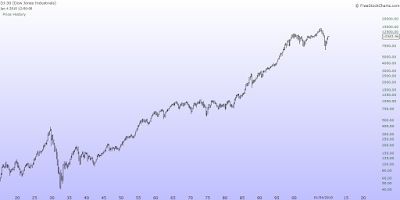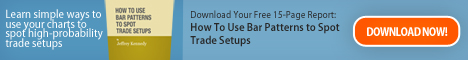## Feb 8, 2014

### Linear or Logarithmic scale?

We all live in an exponential world, and many of the market analyzers use that fact  in their analysis.
The main problem comes from fact, that sometimes analyzers use linear, sometimes logarithmic graph.
You can't use log scale and make linear calculations, and the opposite.
Have a look at the both graphics bellow:At first hand they look totally different, and have nothing common. More observant of you have already noticed, that on both is DJ 30.

Here is an interesting example:

Let's suppose that you have invested \$1000 in General Electric for \$8 per share in 1995, it makes 125 shares. For two years the price rises to \$16 and you cover the long, taking \$8 profit per share in the pocket, which makes 100% profit.
Few years later the price rises to \$30, and you decide to buy your favorite instrument again. But this time you spend \$1000 to buy only 33 shares. However, the price is going higher, reaching \$60 in 2000. Then you decide to sell again, taking profit \$30 per share, which is 100% profit again. Visually the spread between the blue lines is four times wider than the red ones. It's easy to make a mistake, that the second trade's profit is four times bigger. It is so, because the graphic is linear.

Here is the same GE logarithmic scale example:I have calculated GE Fibonacci retracement levels of the move from \$8 to \$60 in both ways:

 Fibo                         38.2% 50% 61.8% Linear                     38.9 33 27.1 Logarithmic       27.21 21.54 17.05

If we follow the linear calculating, could make a conclusion that GE's 61.8%Fibo retrace is nearly (\$27.10)  of its rising up, and as an ewavers using Fibonacci tool to expect bounce up again.
The picture is totally different, if we have calculated that correction in logarithmic way. It is obvious, that 61.8% of the correction is nearly \$17, and there is more dropping down.

The main question is:  Which scale to use?

•  If we are daytraders, can use both, because the difference  is very small.
•  If we are swing or longer term traders or investors- the linear one.

P.S. The prices now differs , I just use an example graphs.

## Feb 7, 2014

### SP500 Update

Most of the EU and US Indexes met key levels and did the "unexpected" very sharp bounce (SP500) from 50% Fibo level of potential  Minor1st of Intermediate 5th of Primary 3.

Next chart is the SP500 volatility and as we see we'll get back on the bull trend.
Good luck to all.## Feb 5, 2014

### SP 500

Medium term SP500 might found support at 50% Fibo retracement of potential Minor first of Intermediate fifth of Primary third wave sinse 3/6/2009. The price could drop to lower  \$1710 level and hit 61.8% Fibo.

This suggesting is based on the Fibonacci extention sequence, i.e. every third wave in this bull market unfolds to 161.8% Fibo of the first wave. If we follow this ratio then Primary wave three target should be far and away from here.

## Dec 6, 2012

### S&P 500

I suppose the last move down to correct at least 38.2% Fibo of this rally, which has started at 16-Nov-2012 and finished at 3-Dec-2012.
I've labeled 1403.74 as minute A wave down, 1415.22 as minute wave B up, and would expect C wave down to unfold. I have labeled B wave as Expanded Flat.

Short term resistance remains 1415.22, and then 1424.81. Short term support remains 1397.57. If the price goes up from here and overcomes the resistance at 1424.81 I have to negate this count, in this case 1397.57 should be Minor wave 2.
-->